# 3. A student used a medicine dropper, instead of a toothpick, to spot the standard 4-cation solutions on the chromat...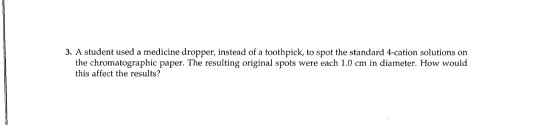3. A student used a medicine dropper, instead of a toothpick, to spot the standard 4-cation solutions on the chromatographic paper. The resulting original spots were each 1.0 cm in diameter. How would this affect the results?

If medicine dropper is used instead of toothpick to spot the standard 4 -cation solutions on the chromatographic paper. The resulting original spots were each 1.0 cm in diameter.

The spot size should not be larger than 1 mm in diameter.

The effect on the results due to this spot diameter leads to poor separation. This is due to the over large spot that cause overlapping of other component spots with similar retention factor (Rf) values.

If this happens then the components cannot be resolved.

##### Add Answer of: 3. A student used a medicine dropper, instead of a toothpick, to spot the standard 4-cation solutions on the chromat...
Similar Homework Help Questions
• ### A student used solutions of aqueous ammonia and hydrochloric acid to make a pure sample of ammonium chloride crystals

A student used solutions of aqueous ammonia and hydrochloric acid to make a pure sample of ammonium chloride crystals. Describe how she should do this.The uestion is on a GCSE higher tier paper and is worth 3 marks.

• ### 2) In preparing the KHP solution, a student did find a 100 ml volumetric flask and instead used a 200 mL size to di...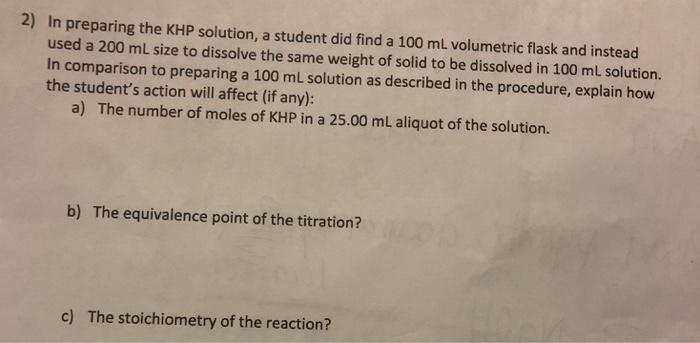2) In preparing the KHP solution, a student did find a 100 ml volumetric flask and instead used a 200 mL size to dissolve the same weight of solid to be dissolved in 100 mL solution. In comparison to preparing a 100 mL solution as described in the procedure, explain how the student's action will affect (if any): a) The number of moles of KHP in a 25.00 mL aliquot of the solution. b) The equivalence point of the titration?...

• ### A student, rushing to get done with the dilutions added 3 ml of NH3 to volumetric flask instead...

1- A student, rushing to get done with the dilutions added 3 ml of NH3 to volumetric flask instead of the required 2 ml. After diluting with water to the 50-mlmark an absorbance reading was taken. sholud this absorbance value be used? Explain your answer2- A student unfamiliar with the use of pipets incorrectly transferred the less than 5.00 ml of Cu(NO3)2 solution into the flask for the first dilution. Nocorrection was made for this mistake. what effect will this...

• ### 1. Intravenous, or IV solutions used in medicine must exert the same osmotic pressure as blood to prevent a net flow of...

1. Intravenous, or IV solutions used in medicine must exert the same osmotic pressure as blood to prevent a net flow of water into or out of the blood cells. The proper concentration for an intravenous NaCl solution is 0.90 g NaCl per 100. mL of water (sometimes refered to as 0.90% m/v). If the van\'t Hoff factor of NaCl is i = 1.8, what is the osmotic pressure of blood at body temperature, 37

• ### 5. The same procedure used in this lab can also be used to separate a 3-component mixture of benz...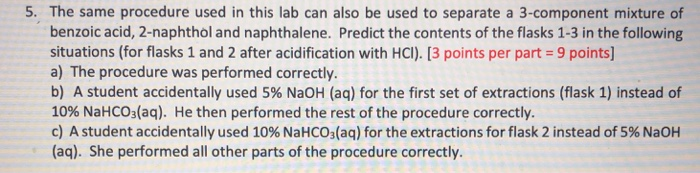Referring to liquid-liquid separation 5. The same procedure used in this lab can also be used to separate a 3-component mixture of benzoic acid, 2-naphthol and naphthalene. Predict the contents of the flasks 1-3 in the following situations (for flasks 1 and 2 after acidification with HCI). [3 points per part 9 points] a) The procedure was performed correctly. b) A student accidentally used 5% NaOH (aq) for the first set of extractions (flask 1) instead of 10% NaHCO3(aq). He...

• ### 2. If two different compounds have the same Rr value, how might they be identified using paper chromatography?...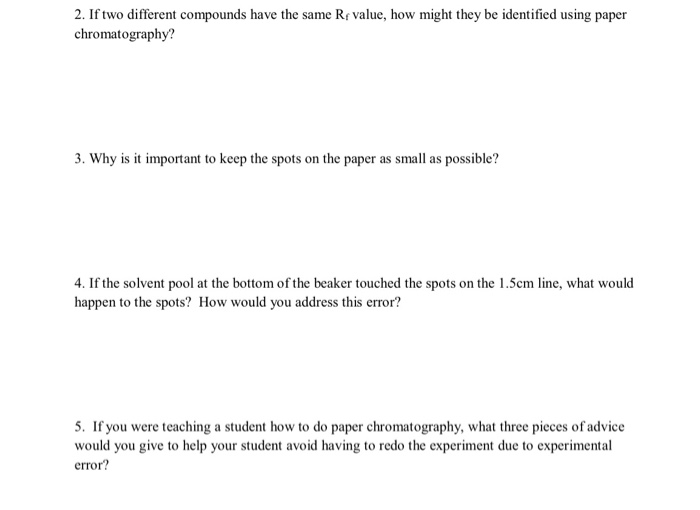2. If two different compounds have the same Rr value, how might they be identified using paper chromatography? 3. Why is it important to keep the spots on the paper as small as possible? 4. If the solvent pool at the bottom of the beaker touched the spots on the 1.5cm line, what would happen to the spots? How would you address this error? 5. If you were teaching a student how to do paper chromatography, what three pieces of...

• ### If we know that the length of time it takes a college student to find a parking spot in the library parking lot follows a normal distribution with a mean of 3.5 minutes and a standard deviation of 1 minute, find the point in the distribution in which 75.8

If we know that the length of time it takes a college student to find a parking spot in the library parking lot follows a normal distribution with a mean of 3.5 minutes and a standard deviation of 1 minute, find the point in the distribution in which 75.8% of the college students exceed when trying to find a parking spot in the library parking lot. A. 2.8 minutes B. 3.2 minutes C. 3.4 minutes D. 4.2 minutes

• ### The correct answer is 7.14x10^-4, but I am not sure how to calculate it. The method of standard additions was used to de...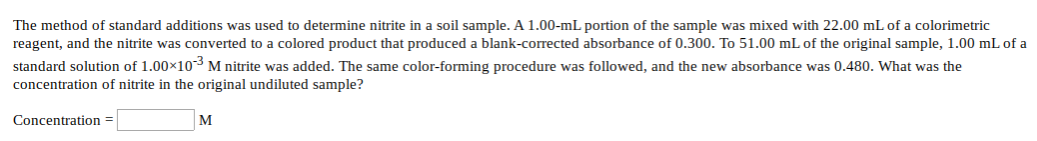The correct answer is 7.14x10^-4, but I am not sure how to calculate it. The method of standard additions was used to determine nitrite in a soil sample. A 1.00-mL portion of the sample was mixed with 22.00 mL of a colorimetric reagent, and the nitrite was converted to a colored product that produced a blank-corrected absorbance of 0.300. To 51.00 mL of the original sample, 1.00 mL of a standard solution of 1.00x10 M nitrite was added. The same...

• ### The method of standard additions was used to determine nitrite in a soil sample. A 1.00-ml portion of the sample was mi...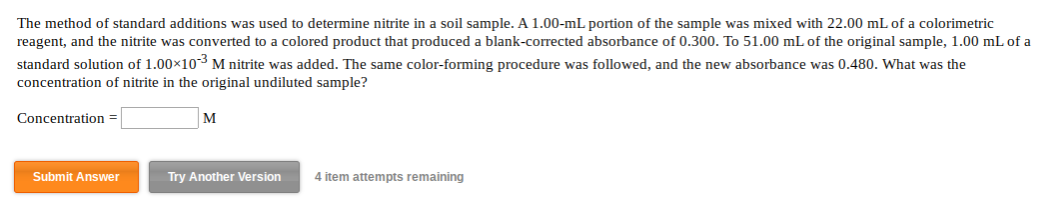The method of standard additions was used to determine nitrite in a soil sample. A 1.00-ml portion of the sample was mixed with 22.00 mL of a colorimetric reagent, and the nitrite was converted to a colored product that produced a blank-corrected absorbance of 0.300. To 51.00 mL of the original sample, 1.00 mL of a standard solution of 1.00×10 M nitrite was added. The same color-forming procedure was followed, and the new absorbance was 0.480. What was the concentration...

• ### ) In the figure, a slit 0.30 mm wide is illuminated by light of wavelength 426 nm. A diffraction attern is seen on a screen 2.8 m from the slit. What is the linear distance on the screen betw...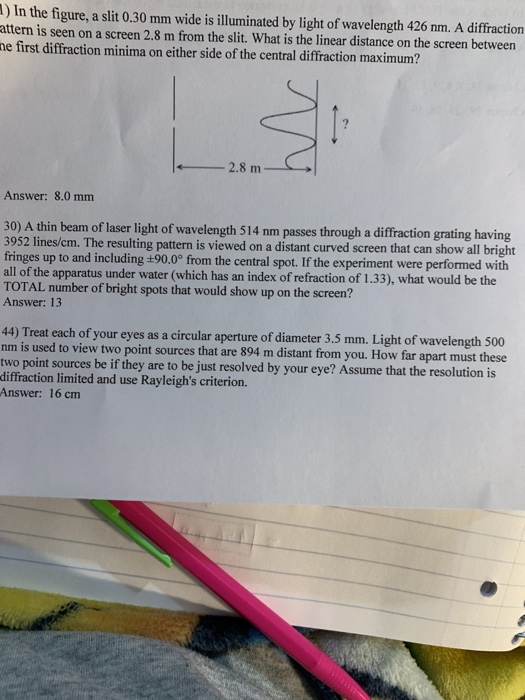) In the figure, a slit 0.30 mm wide is illuminated by light of wavelength 426 nm. A diffraction attern is seen on a screen 2.8 m from the slit. What is the linear distance on the screen between e first diffraction minima on either side of the central diffraction maximum? Answer: 8.0 mm 30) A thin beam of laser light of wavelength 514 nm passes through a diffraction grating having 3952 lines/cm. The resulting pattern is viewed on a...

Need Online Homework Help?